# Python Matplotlib – An Overview

It is a very powerful plotting library useful for those working with Python and NumPy. And for making statistical interference, it becomes very necessary to visualize our data and Matplotlib is the tool that can be very helpful for this purpose. It provides MATLAB like interface only difference is that it uses Python and is open source.

## Installation

It is very easy to install Matplotlib in your PC, you just have to open the command prompt and give the following command :

`python -m pip install -U matplotlib`

It is assumed that you are using the latest version of Python i.e Python 3. and if you wish to foray into the world of Machine Learning it is suggested to download Anaconda Package Distribution which comes with many preinstalled libraries like Matplotlib, Numpy, Pandas, Scikit-learn, and most important one is Jupyter Notebook.

## Basics

Before moving further let us clear our basics,

• Figure – It is a whole figure which contains one or more than one axes or plots.
• Axes – A figure contains usually more than one axes (plots) and it may contain two or three in case of three-dimensional structure or objects. Each Axes has a title, an X –label, and Y –label.
• Axis- It takes care of generating graph limits
• Artists- Mostly they are tied to the axes, and whatever we see on the figure like text objects, line2d objects, collection objects.

Now that we have understood the basics, let us make a simple plot:

#### Making a simple plot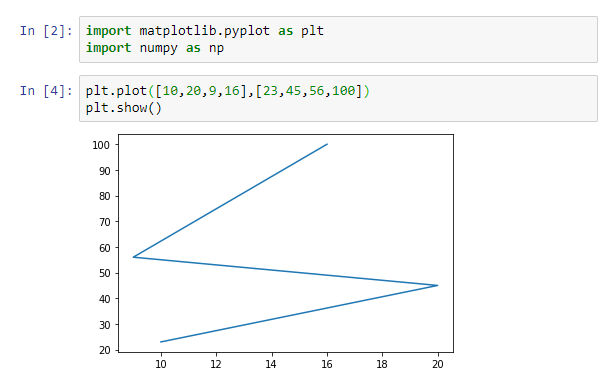So, here we are passing two arrays as input and using `show()`, to get the required plot, as from the graph you can see that the first array appears on the x-axis and the second array appears on the y-axis of the given plot. Now let us add the title to the plots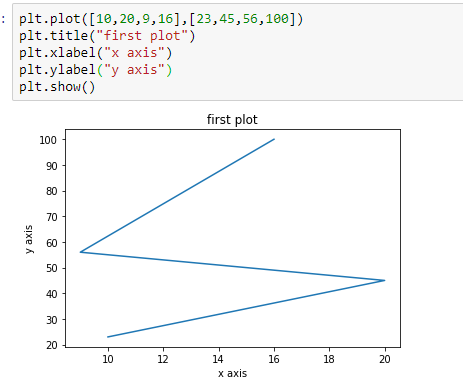#### Plotting Multiple Sets of Data

We can also plot multiple sets of data by passing in multiple sets of arguments of X and Y-axis in the plot,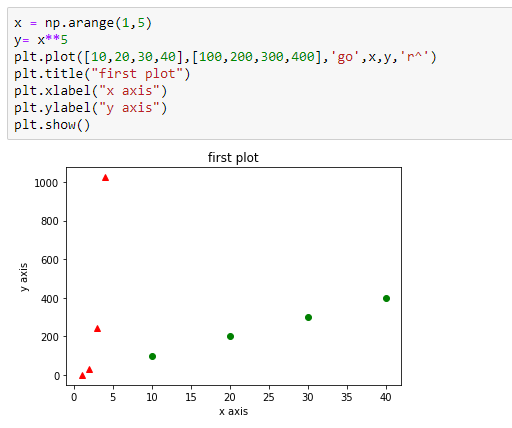#### Plotting with keyword Strings

Sometimes we have data in a format which lets us access the particular variables with string, so matplotlib gives us such object with the data keyword argument. So with these, you can generate plots with strings corresponding to the given variables: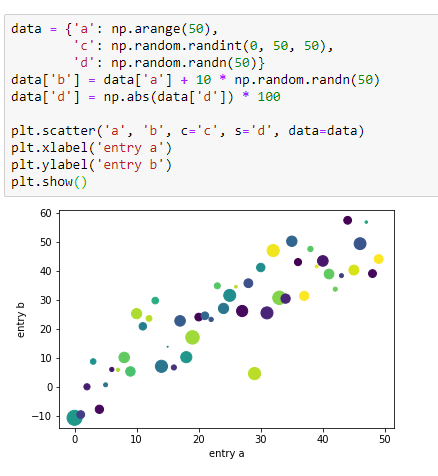#### Working with text

Matplotlib has a `text()` command which can be used to add text in an arbitrary location and the `label()` and `title()`, are used to add text in the predefined positions :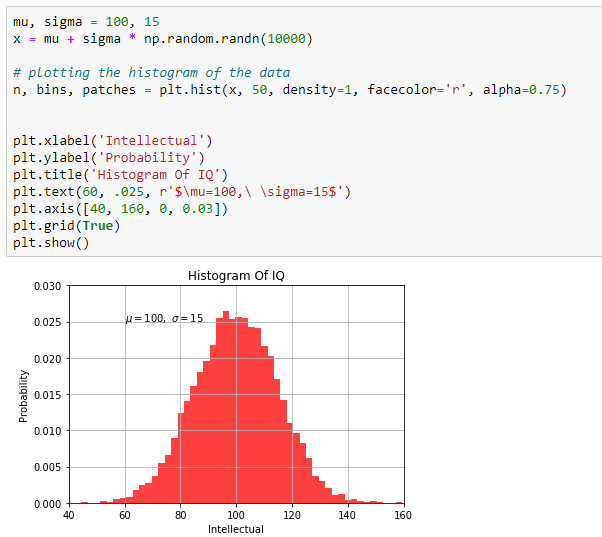## Plotting Bar Graph

Now that we have already learned how to use texts and how to plot a histogram, so let’s move forward and learn how can you plot the bar graph. As we know that the bar graphs are the most common type of graphs, so Matplotlib provides a `bar()`, function to make bar graphs which may take the desired arguments which will we specify,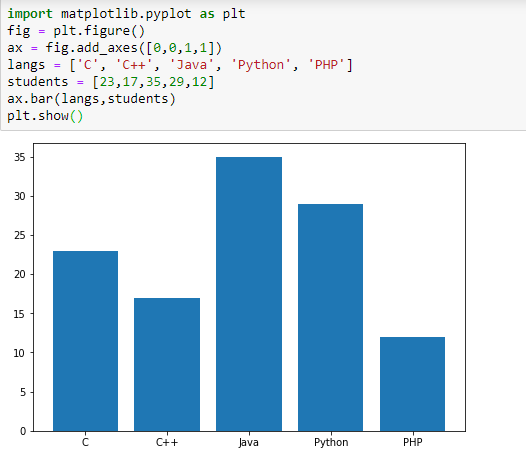#### Plotting Pie charts

We can also plot the pie chart simply by using the `pie()` command and passing the required arguments,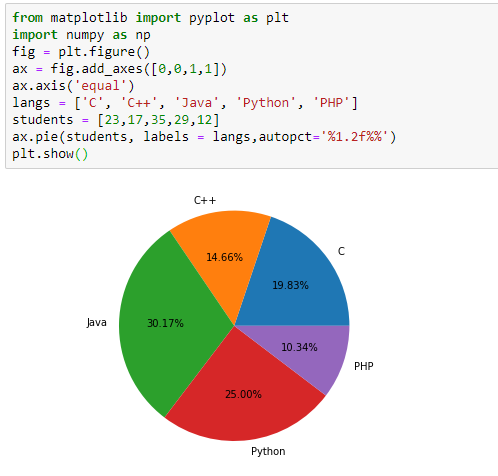#### Scatter and Three Dimensional plotting

It is the most basic and most widely used graphs, especially they are of great use in solving the regression problems. So let’s say we are plotting to scatter diagram of grades range vs grades of boys and girls in two different colors, (we always use different colors as the graph should be easy to visualize)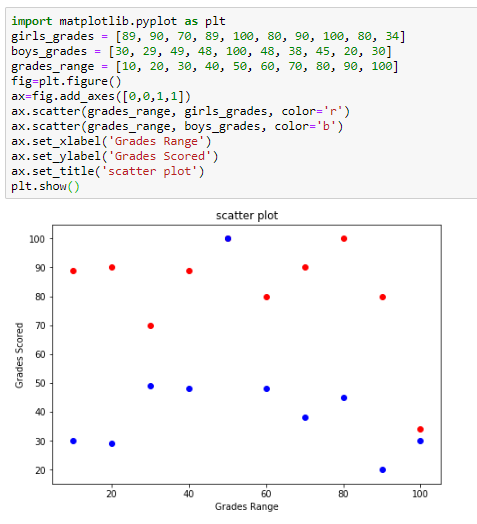And we can also view the above scatter in the three-dimensional view, but for this, we have to import another module called mplot3d, and when the module is imported we have to create three-dimensional axes by using the keyword.

Projection = ‘3d’ to the `axes()`, and when the object is created, we pass our arguments girls and boys grades, and grade range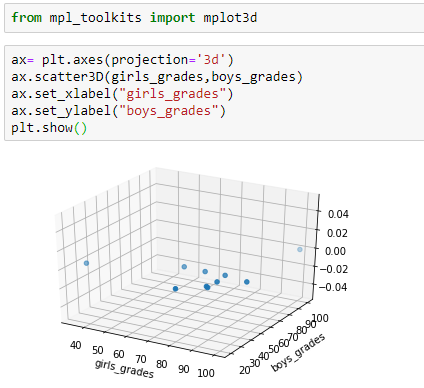## Conclusion

You have gone through today’s Matplotlib tutorial successfully! There is still much to learn, but you’re definitely ready to go out on your own and create your own amazing plots. If you’re eager to discover more from Matplotlib, consider checking out Geeks for Geeks Archives to learn how to work with matplotlib if you’re ready to start exploring interactive data visualizations with Python.

My Personal Notes arrow_drop_upCheck out this Author's contributed articles.

If you like GeeksforGeeks and would like to contribute, you can also write an article using contribute.geeksforgeeks.org or mail your article to contribute@geeksforgeeks.org. See your article appearing on the GeeksforGeeks main page and help other Geeks.

Please Improve this article if you find anything incorrect by clicking on the "Improve Article" button below.

Article Tags :

Be the First to upvote.

Please write to us at contribute@geeksforgeeks.org to report any issue with the above content.